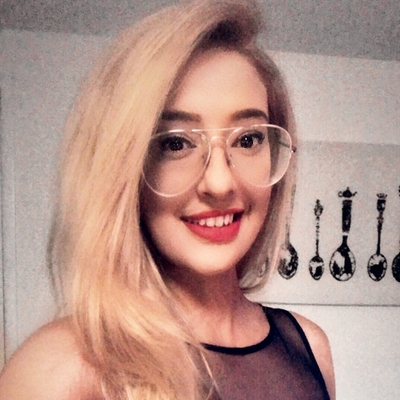# Introduction to Convolutional Neural Networks with Weights & BiasesLavanya Shukla
21
Nov
2019

In this tutorial we'll walk through a simple convolutional neural network to classify the images in the cifar10 dataset.

You can find the accompanying code here. We highly encourage you to fork this notebook, tweak the parameters, or try the model with your own dataset!

# Convolutional Neural Networks

###### Convolution Layer

The convolution layer is made up of a set of independent filters. Each filter slides over the image and creates feature maps that learn different aspects of an image.

Source

###### Convolutional Neural Network

A CNN uses convolutions to connected extract features from local regions of an input. Most CNNs contain a combination of convolutional, pooling and affine layers. CNNs offer fantastic performance on visual recognition tasks, where they have become the state of the art.

###### Pooling

The pooling layer reduce the size of the image representation and so the number of parameters and computation in the network. Pooling usually involves taking either the maximum or average value across the pooled area.

## Creating A Basic Convolutional Neural Network

# Define model
model = tf.keras.models.Sequential()

# Conv2D adds a convolution layer with 32 filters that generates 2 dimensional feature maps to learn different aspects of our image
input_shape=X_train.shape[1:], activation='relu'))

# MaxPooling2D layer reduces the size of the image representation our convolutional layers learnt, and in doing so it reduces the number of parameters and computations the network needs to perform.

# Dropout layer turns off a percentage of neurons at every step

# Flattens our array so we can feed the convolution layer outputs (a matrix) into our fully connected layer (an array)

# Dense layer creates dense, fully connected layers with x inputs and y outputs - it simply outputs the dot product of our inputs and weights

# Compile the model and specify the optimizer and loss function
model.compile(loss='categorical_crossentropy',
metrics=['accuracy'])

# Fit the model to the training data, specify the batch size and the WandbCallback() to track model
model.fit(X_train, y_train, epochs=10, batch_size=128, validation_data=(X_test, y_test),
callbacks=[wandb.keras.WandbCallback(data_type="image", labels=class_names, save_model=False)])

## Convolutional Neural Network with Data Augmentation

Data augmentation artificially expands the training dataset by creating slightly modified versions of images in the dataset - by scaling, shifting and rotating the images in the training set.

# Define the model (same as above)
...

# Compile the model
model.compile(loss='categorical_crossentropy',
metrics=['accuracy'])

datagen = ImageDataGenerator(
featurewise_center=False,  # set input mean to 0 over the dataset
samplewise_center=False,  # set each sample mean to 0
featurewise_std_normalization=False,  # divide inputs by std of the dataset
samplewise_std_normalization=False,  # divide each input by its std
zca_whitening=False,  # apply ZCA whitening
rotation_range=15,  # randomly rotate images in the range (degrees, 0 to 180)
width_shift_range=0.1,  # randomly shift images horizontally (fraction of total width)
height_shift_range=0.1,  # randomly shift images vertically (fraction of total height)
horizontal_flip=True,  # randomly flip images
vertical_flip=False)  # randomly flip images
datagen.fit(X_train)

# Fit the model on the batches generated by datagen.flow()
model.fit_generator(datagen.flow(X_train, y_train,
batch_size=config.batch_size),
steps_per_epoch=X_train.shape // config.batch_size,
epochs=config.epochs,
validation_data=(X_test, y_test),
callbacks=[WandbCallback(data_type="image", labels=class_names)])

# Visualize Predictions Live

## Project Overview

1. Check out the project page to see your results in the shared project.
2. Press 'option+space' to expand the runs table, comparing all the results from everyone who has tried this script.
3. Click on the name of a run to dive in deeper to that single run on its own run page.

## Visualize Performance

Click through to a single run to see more details about that run. For example, on this run page you can see the performance metrics I logged when I ran this script.

## Review Code

The overview tab picks up a link to the code. In this case, it's a link to the Google Colab. If you're running a script from a git repo, we'll pick up the SHA of the latest git commit and give you a link to that version of the code in your own GitHub repo.

## Visualize System Metrics

The System tab on the runs page lets you visualize how resource efficient your model was. It lets you monitor the GPU, memory, CPU, disk, and network usage in one spot.

## Next Steps

As you can see running sweeps is super easy! We highly encourage you to fork this notebook, tweak the parameters, or try the model with your own dataset!

## More about Weights & Biases

We're always free for academics and open source projects. Email carey@wandb.com with any questions or feature suggestions. Here are some more resources:

1. Documentation - Python docs
2. Gallery - example reports in W&B
3. Articles - blog posts and tutorials